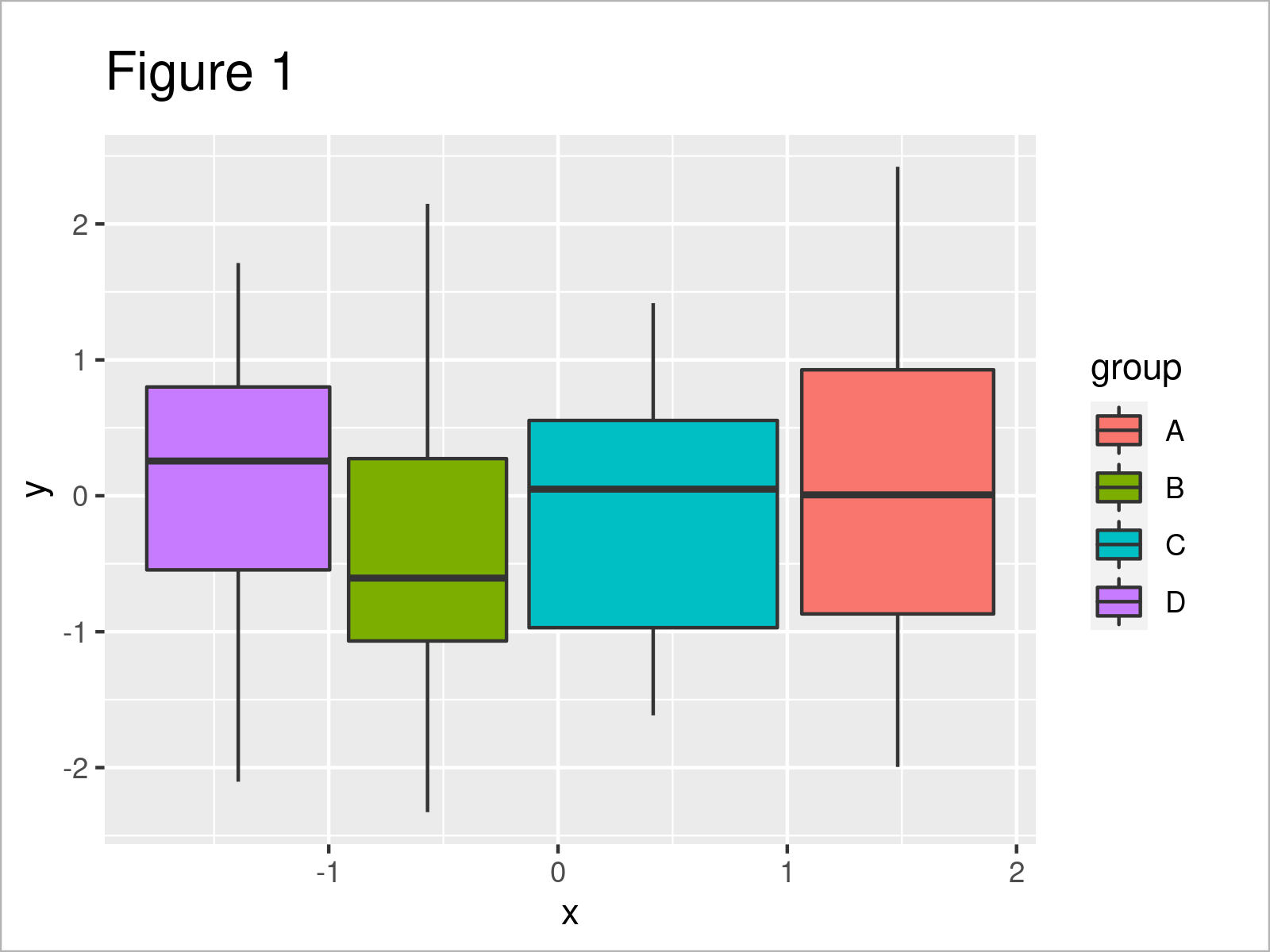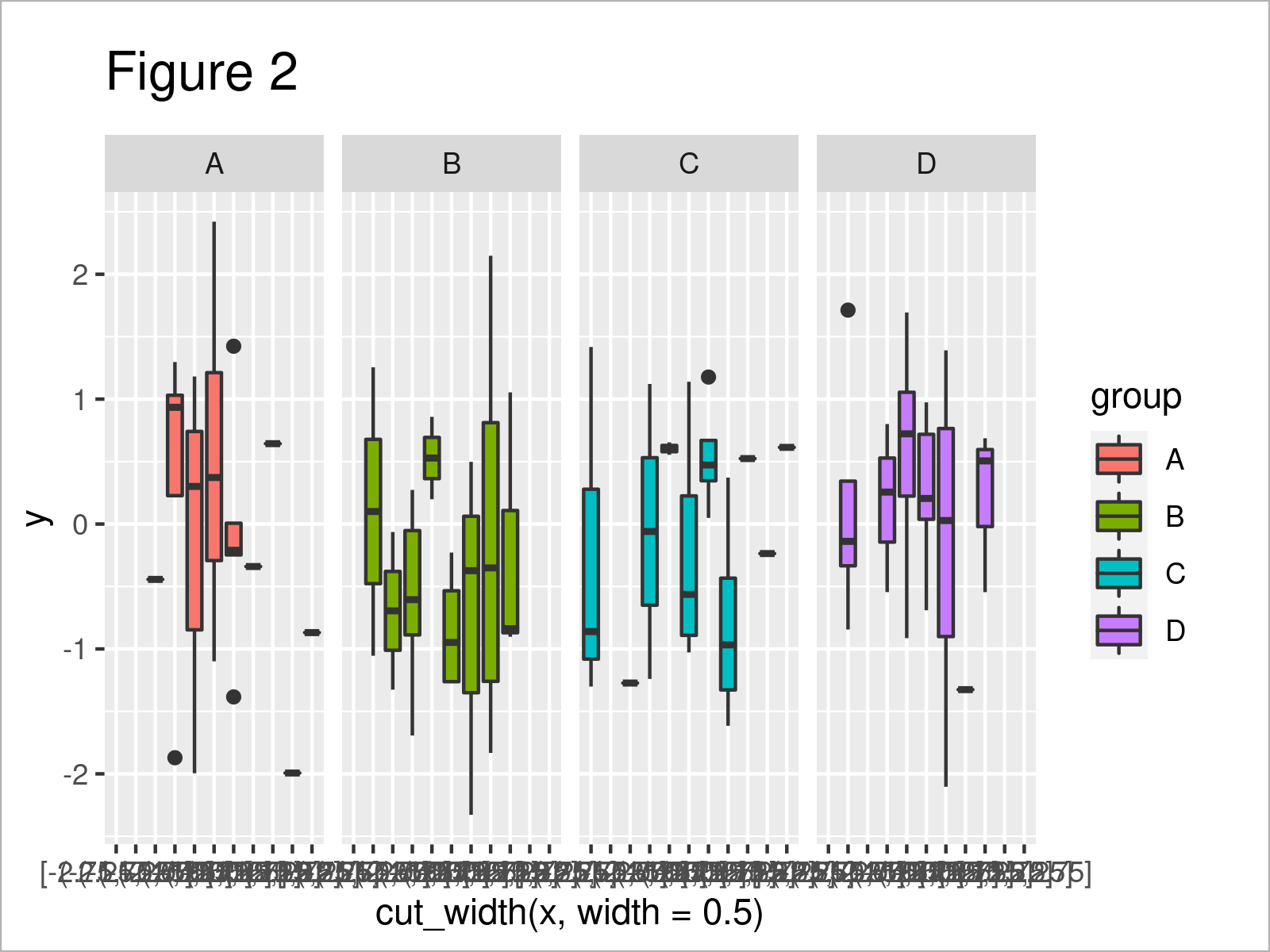# Arrange Boxplot of Multiple Y-Variables for Groups of Continuous X in R (Example)

In this R tutorial you’ll learn how to split a continuous variable into boxplot-groups.

Sound good? Let’s dive right in…

## Example Data, Packages & Basic Graphic

The first step is to create some example data:

```set.seed(354967)                     # Create example data frame
data <- data.frame(x = rnorm(100),
y = rnorm(100),
group = rep(LETTERS[1:4], each = 25))As you can see based on Table 1, the exemplifying data is a data frame consisting of three columns called “x”, “y”, and “group”. The variables x and y have the numeric data class and the variable group is a character.

Note that our data is in long format. In case your data is in wide format, you may transform it to long format first.

For the following R syntax, we also have to install and load the ggplot2 package:

```install.packages("ggplot2")          # Install & load ggplot2
library("ggplot2")```

Now, we can draw the data as follows:

```ggplot(data,                         # Draw ggplot2 boxplot by group
aes(x = x,
y = y,
fill = group)) +
geom_boxplot()```The output of the previous R programming code is shown in Figure 1 – We have created a ggplot2 boxplot containing a separate box for each of our four groups.

## Example: Split Continuous x-Variable into Boxplot Groups

The following R syntax shows how to separate a continuous x-variable in each box of a boxplot into multiple subgroups.

To split the continuous x-variable, we can use the cut_width function of the ggplot2 package.

Furthermore, we can assign each main group to its own facet using the facet_grid function. This helps to differentiate between the main groups.

Consider the following R code:

```ggplot(data,                         # Draw facet_grid boxplot by split groups
aes(x = cut_width(x, width = 0.5),
y = y,
fill = group)) +
geom_boxplot() +
facet_grid(. ~ group)```After executing the previous R syntax the ggplot2 boxplot with four facets and several subgroups within each facet shown in Figure 2 has been plotted.

Note that the x-axis labels of this plot are a bit messed up. You may change that according to your needs using the scale_x_discrete function.

## Video & Further Resources

I have recently released a video on the Statistics Globe YouTube channel, which demonstrates the examples of this page. You can find the video below:

Please accept YouTube cookies to play this video. By accepting you will be accessing content from YouTube, a service provided by an external third party.If you accept this notice, your choice will be saved and the page will refresh.

In addition, you could have a look at the other tutorials on my homepage:

You have learned in this post how to divide a continuous variable into boxplot-groups in R programming. Please let me know in the comments below, if you have additional questions.

Subscribe to the Statistics Globe Newsletter